# RD Sharma Solutions for Class 6 Maths Chapter 17: Symmetry Objective Type Questions

This exercise consists of problems which cover the concepts of the entire Chapter. These solutions mainly help students self-analyse their knowledge about the Chapter. The exercise-wise problems are solved by the faculty at BYJU’S after researching on each concept. It helps the students to improve their problem solving and logical thinking abilities which are important from the exam point of view. Scoring good marks in the exam is made easy with the help of RD Sharma Solutions Class 6 Maths Chapter 17 Symmetry Objective Type Questions PDF which are available here.

## RD Sharma Solutions for Class 6 Maths Chapter 17: Symmetry Objective Type Questions Download PDF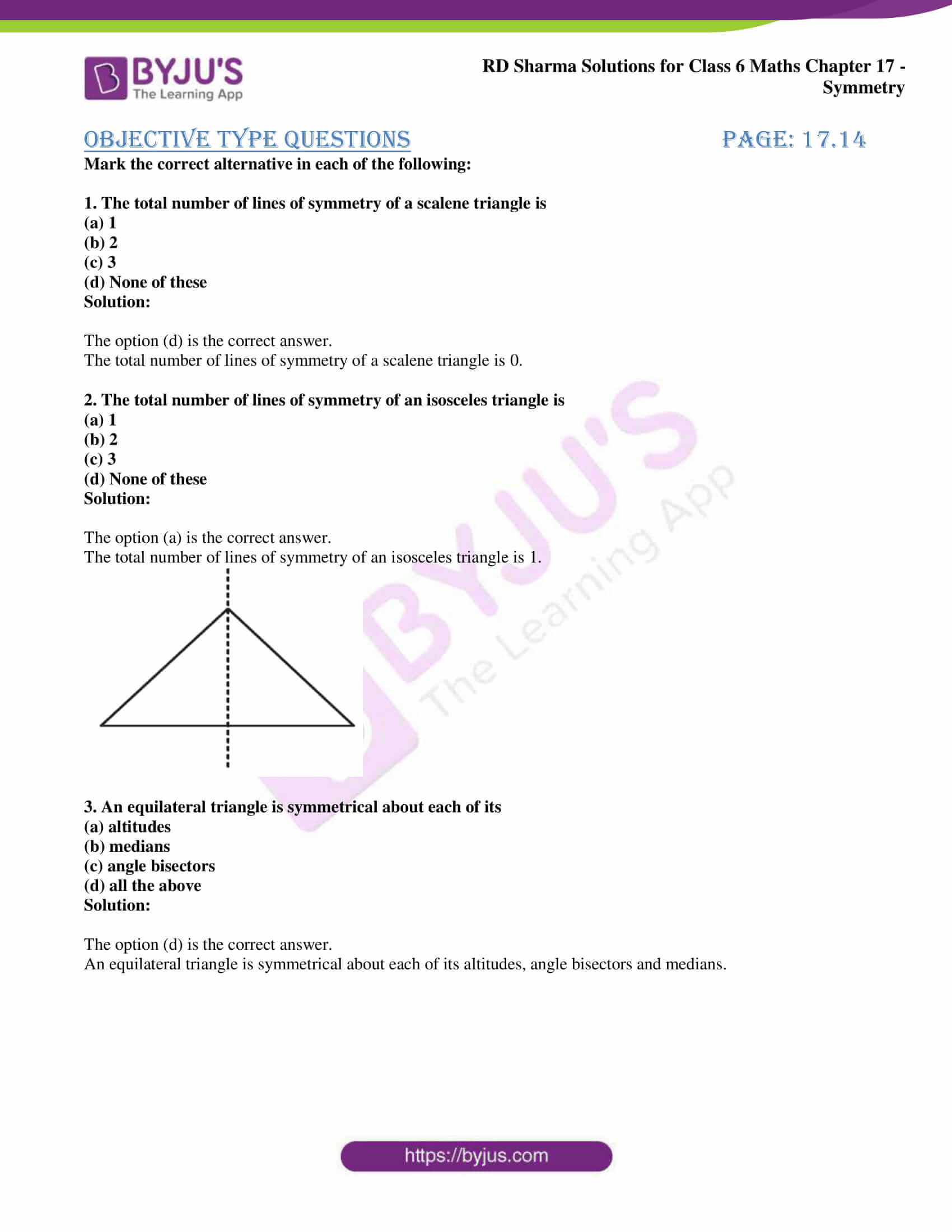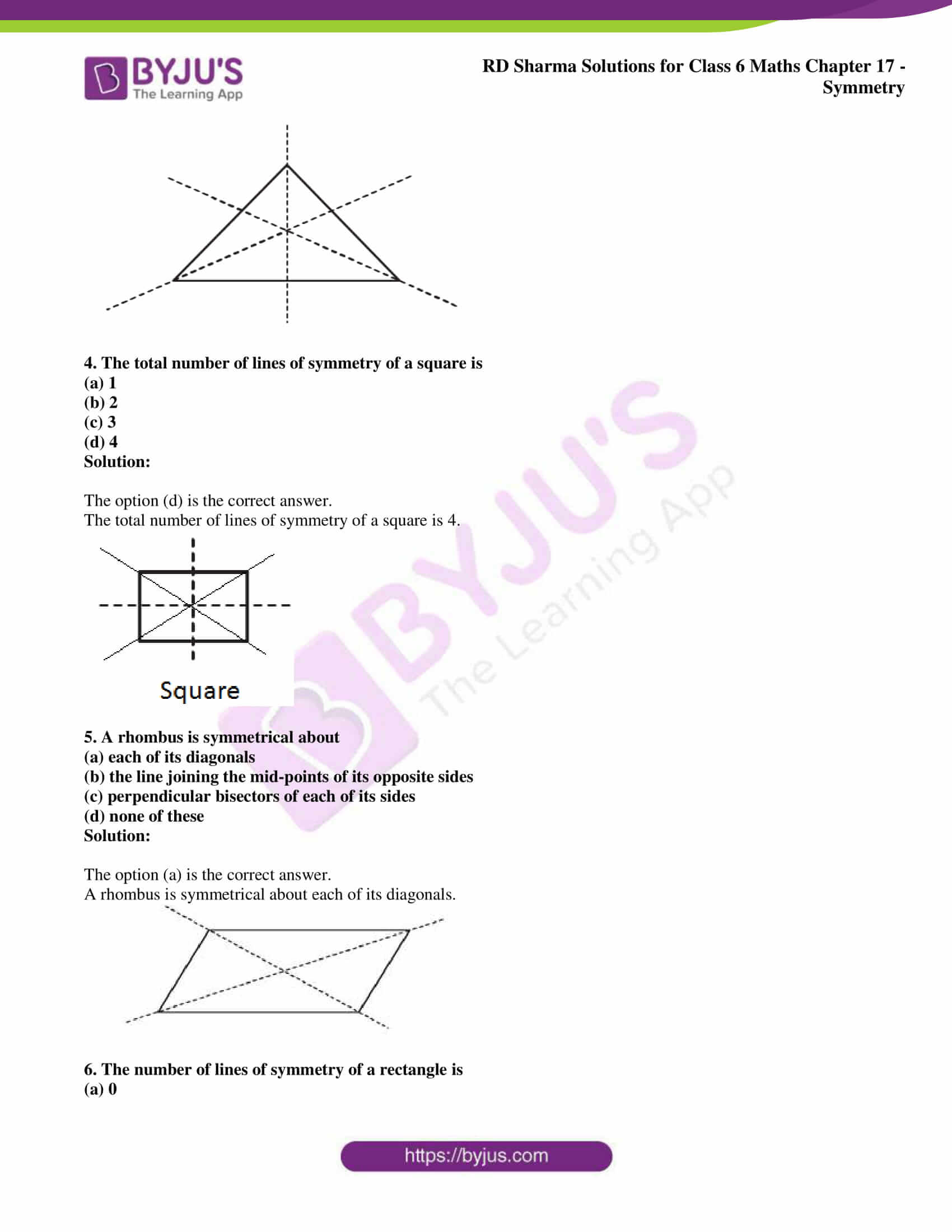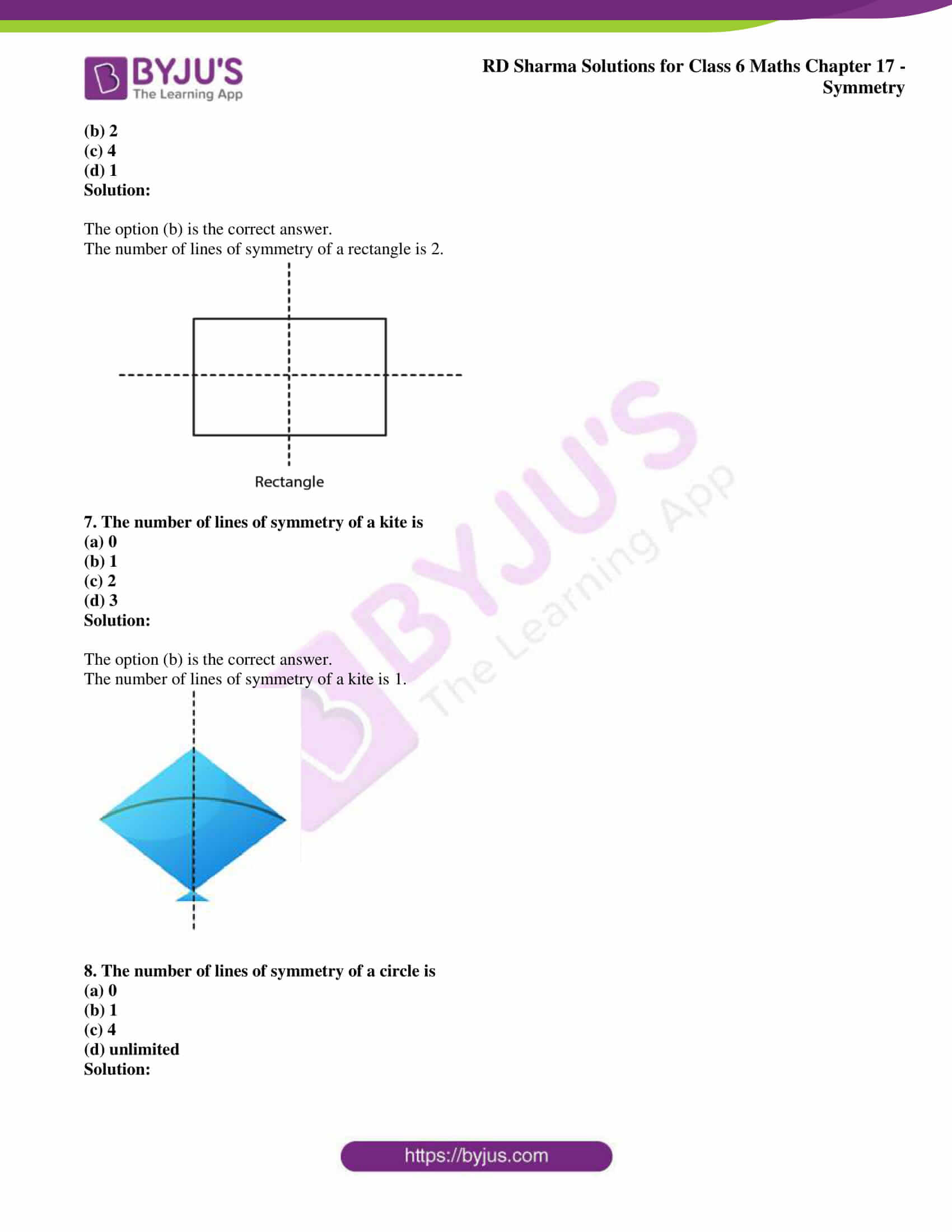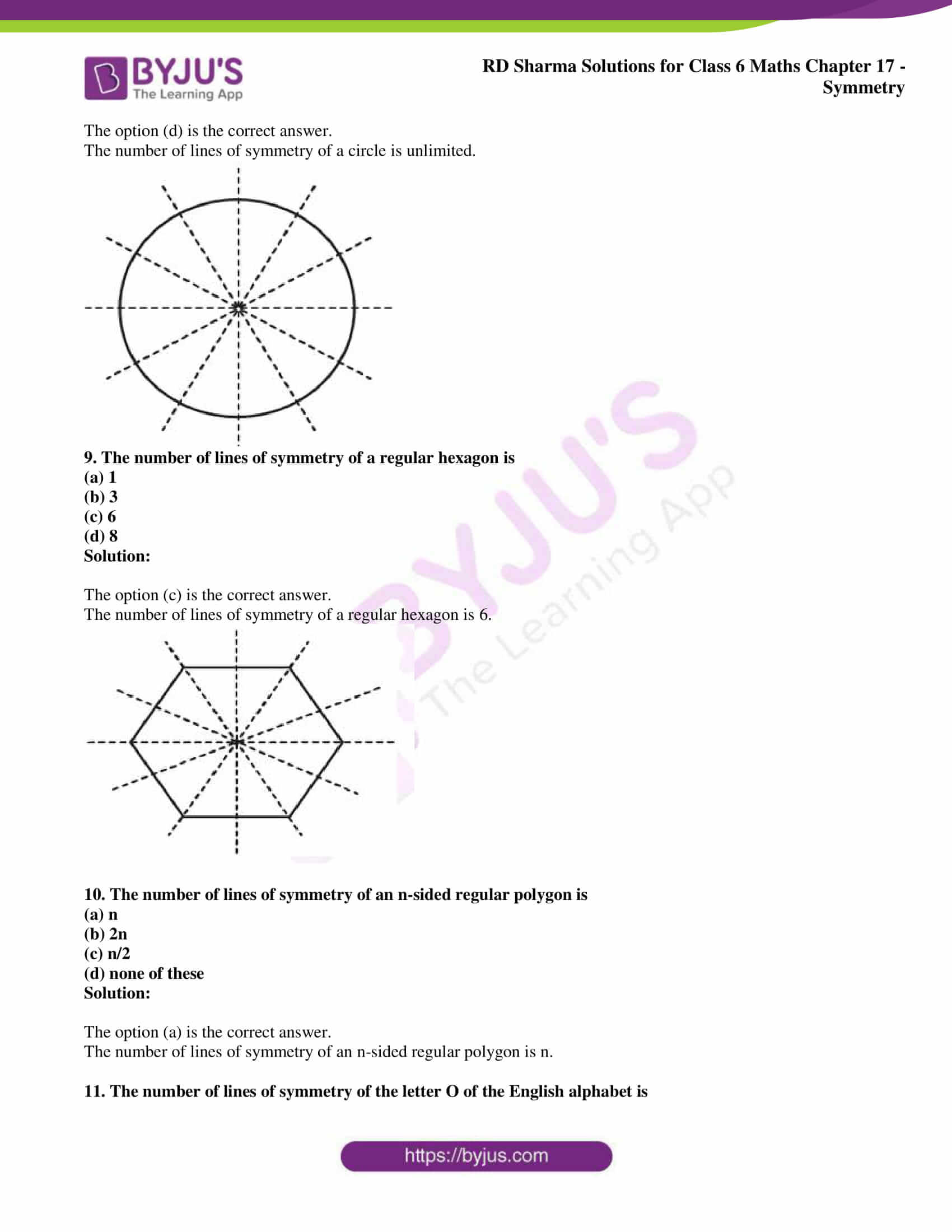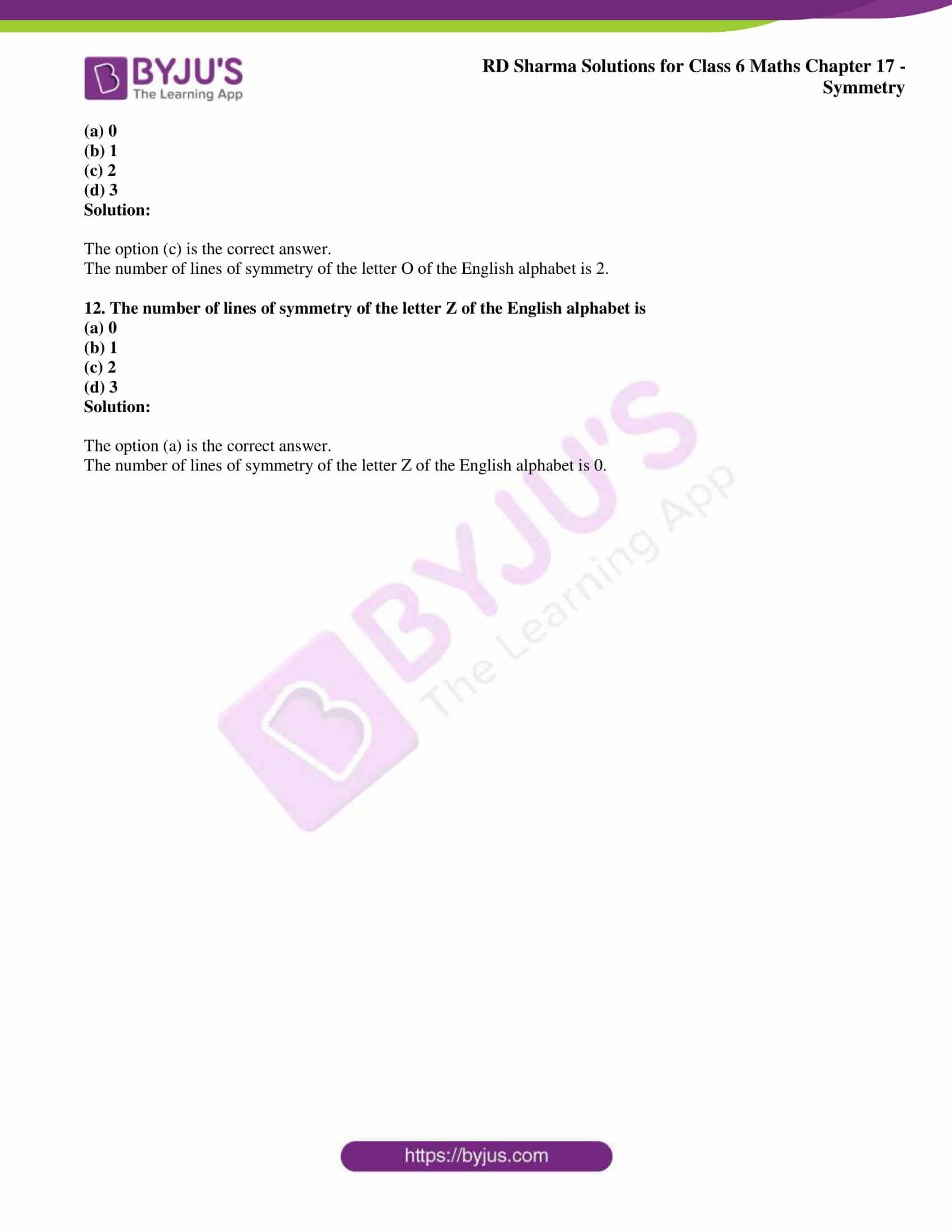### Objective Type Questions page: 17.14

Mark the correct alternative in each of the following:

1. The total number of lines of symmetry of a scalene triangle is
(a) 1
(b) 2
(c) 3
(d) None of these

Solution:

The option (d) is the correct answer.

The total number of lines of symmetry of a scalene triangle is 0.

2. The total number of lines of symmetry of an isosceles triangle is
(a) 1
(b) 2
(c) 3
(d) None of these

Solution:

The option (a) is the correct answer.

The total number of lines of symmetry of an isosceles triangle is 1.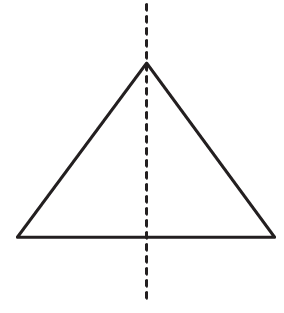3. An equilateral triangle is symmetrical about each of its
(a) altitudes
(b) medians
(c) angle bisectors
(d) all the above

Solution:

The option (d) is the correct answer.

An equilateral triangle is symmetrical about each of its altitudes, angle bisectors and medians.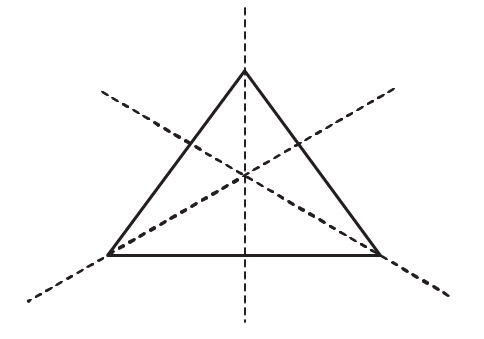4. The total number of lines of symmetry of a square is
(a) 1
(b) 2
(c) 3
(d) 4

Solution:

The option (d) is the correct answer.

The total number of lines of symmetry of a square is 4.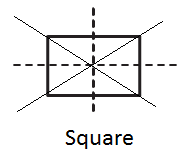5. A rhombus is symmetrical about
(a) each of its diagonals
(b) the line joining the mid-points of its opposite sides
(c) perpendicular bisectors of each of its sides
(d) none of these

Solution:

The option (a) is the correct answer.

A rhombus is symmetrical about each of its diagonals.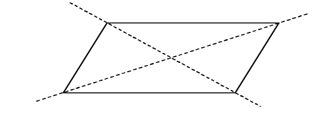6. The number of lines of symmetry of a rectangle is
(a) 0
(b) 2
(c) 4
(d) 1

Solution:

The option (b) is the correct answer.

The number of lines of symmetry of a rectangle is 2.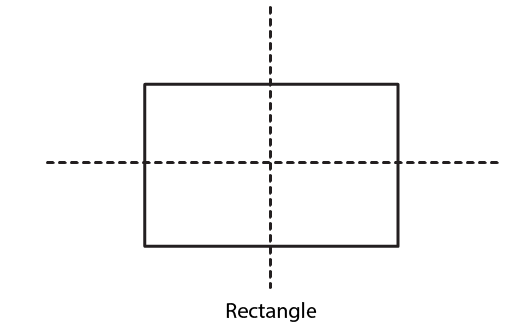7. The number of lines of symmetry of a kite is
(a) 0
(b) 1
(c) 2
(d) 3

Solution:

The option (b) is the correct answer.

The number of lines of symmetry of a kite is 1.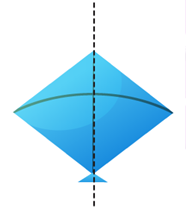8. The number of lines of symmetry of a circle is
(a) 0
(b) 1
(c) 4
(d) unlimited

Solution:

The option (d) is the correct answer.

The number of lines of symmetry of a circle is unlimited.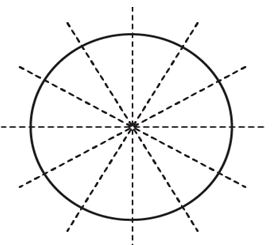9. The number of lines of symmetry of a regular hexagon is
(a) 1
(b) 3
(c) 6
(d) 8

Solution:

The option (c) is the correct answer.

The number of lines of symmetry of a regular hexagon is 6.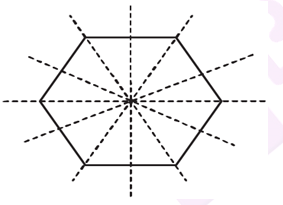10. The number of lines of symmetry of an n-sided regular polygon is
(a) n
(b) 2n
(c) n/2
(d) none of these

Solution:

The option (a) is the correct answer.

The number of lines of symmetry of an n-sided regular polygon is n.

11. The number of lines of symmetry of the letter O of the English alphabet is
(a) 0
(b) 1
(c) 2
(d) 3

Solution:

The option (c) is the correct answer.

The number of lines of symmetry of the letter O of the English alphabet is 2.

12. The number of lines of symmetry of the letter Z of the English alphabet is
(a) 0
(b) 1
(c) 2
(d) 3

Solution:

The option (a) is the correct answer.

The number of lines of symmetry of the letter Z of the English alphabet is 0.